# How to begin modelling with ASCEND

This is an attempt at helping beginners learn ASCEND. Art has started the page that will walk through the process of developing a reusable model for a tank. I am experimenting with adding equations (in latex) and code and scripts to see how unwieldy it could get (The objective is to keep it as simple as possible and not too intimidating to a beginner, yet highlight important issues on how to model correctly. We should provide links on this page to the models that can be downloaded and run). I will be working on cubic equations of state.

## Collect equations you need for the model

Note that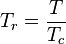$T_r = \frac{T}{T_c}$ where Tc is the critical temperature and Tr is the reduced temperature and similarly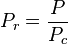$P_r = \frac{P}{P_c}$ where Pc is the critical pressure and Pr is the reduced pressure

### Generic cubic Equations of State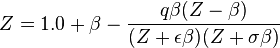$Z = 1.0 + \beta - \frac{q \beta (Z - \beta)}{(Z + \epsilon \beta)(Z + \sigma\beta)}$

#### Setting parameters to get PengRobinson, Redlich Kwong, Soave Redlich Kwong and VdW

##### Pengrobinson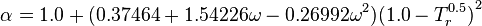$\alpha = {1.0 + (0.37464+1.54226\omega-0.26992\omega^2)(1.0-T_r^{0.5})}^2$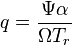$q = \frac{\Psi \alpha}{\Omega T_r}$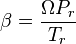$\beta = \frac{\Omega P_r}{T_r}$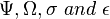$\Psi, \Omega, \sigma~and~\epsilon$ are constants and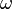$~\omega~$ is the Pitzer acentric factor

## Translate those equations into the ASCEND language

PSI :== 0.42748;
OMEGA :== 0.07780;
sigma :== 2.414213562;

eps :== 0.414213562;
Tr = T/Tc;
Pr = P/Pc;

alpha = (1.0 + (0.37464+1.54226*omega-0.26992*omega^2)*(1.0-Tr^(0.5)))^2;

q = PSI*alpha/(OMEGA*Tr);
beta = OMEGA*Pr/Tr;

Z = 1.0 + beta - q*beta*(Z - beta)/((Z + eps*beta)*(Z + sigma*beta));


## Write METHODS that will make it easy to read the MODELS

ASCEND comes with several METHODS that are executed automatically. ClearAll, default_self, values and specify for example. The objective is to set the system to be square, so you have a solution - that is, the number of unknowns should equal the number of independent equations.

## Thermodynamic Models that come with ASCEND

Rackett, UNIFAC, WILSON, Ideal

### How to call and use the MODELS

Show how those MODELS set variables and METHODS